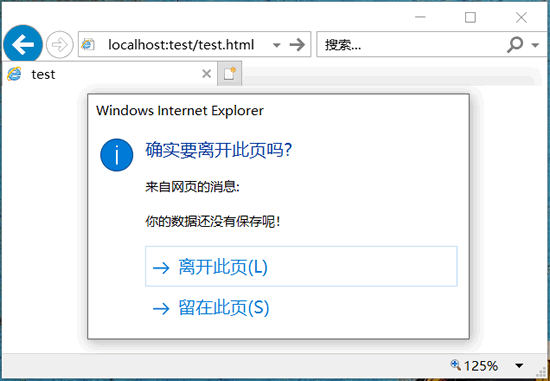• 内容
• 评论
• 相关

## 页面初始化

load 事件类型在页面完全加载完毕的时候触发。该事件包含所有的图形图像、外部文件（如 CSS、JS 文件等）的加载，也就是说，在页面所有内容全部加载之前，任何 DOM 操作都不会发生。为 window 对象绑定 load 事件类型的方法有两种。

1) 直接为 window 对象注册页面初始化事件处理函数。

```window.onload = f;
function f() {
}```

2) 在页面 <body> 标签中定义 onload 事件处理属性。

```<body onload="f()">
<script>
function f() {
}
</script>```

#### 示例1

```<body onload="f()">
<script>
function f() {
}
</script>
</body>```

#### 示例2

```<body onload="f1()">
<script>
function f1 () {
}
function f2 () {
}
</script>
</body>```

1) 在 body 元素中通过事件属性的形式回调函数。

```<body onload="f('Hi')">
<script>
function f (a) {
}
</script>
</body>```

2) 通过函数嵌套或闭包函数来实现。

```window.onload = function () {  //事件处理函数
f("Hi");  //调用函数
}
functioin f(a) {  //被处理函数
}```

```window.onload = f("Hi");
function f(a) {
return function () {
}
}```

```window.onload = function () {
f1();  //绑定响应函数1
f2();  //绑定响应函数2
}
function f1() {
}
function f2() {
}```

```window.onload = f1();
function f1(){
}
function f2() {
}```

```if (window.addEventListener) {  //兼容DOM标准
} else {  //兼容IE事件模型
}```

## 结构初始化

#### 示例1

```<script>
}
function f () { alert("我要提前执行了"); }
function f1 () { alert("页面初始化完毕"); }
</script>
<img src="Winter.jpg">```

#### 示例2

```if (window.ActiveXObject) {  //兼容IE事件模型
document.write ("<script id=ie_onload defer src=javascript:void(0)><\/script");  //写入脚本标签
//判断脚本标签的状态
if (this.readyState == "complete") {  //如果状态为完成，则说明文档结构加载已完毕
this.onreadystatechange = null;  //清空当前反腐
f();  //调用预先执行的回调函数
}
}
}```

<script> 标签在文档结构加载完毕之后才加载，于是，只要判断它的状态就可以确定当前文档结构是否已经加载完毕并触发响应的事件。

#### 示例3

```if (/WebKit/i.test(navigator.userAgent)) {  //兼容Safari浏览器
var _timer = setInterval (function () {  //定义时间监测器
clearInterval(_timer);  //清空时间监测器
f();  //调用预先执行的回调函数
}
}, 10);
}```

## 页面卸载

#### 示例

```window.onunload = f;
function f() {
}```

```window.beforeunload = function (e) {
return "您的数据还没有保存！";
}```0条评论